Defining the IRR

IRR is a rate of return used in capital budgeting to measure and compare the profitability of investments; the higher IRR, the more desirable the project.

Learning Objectives

Explain how Internal Rate of Return is used in capital budgeting

Key Takeaways

Key Points

• The IRR of an investment is the discount rate at which the net present value of costs (negative cash flows ) of the investment equals the net present value of the benefits (positive cash flows) of the investment.
• The higher a project’s IRR, the more desirable it is to undertake the project.
• A firm (or individual) should, in theory, undertake all projects or investments available with IRRs that exceed the cost of capital. Investment may be limited by availability of funds to the firm and/or by the firm’s capacity or ability to manage numerous projects.

Key Terms

• effective interest rate: The effective interest rate, effective annual interest rate, annual equivalent rate (AER), or simply effective rate is the interest rate on a loan or financial product restated from the nominal interest rate as an interest rate with annual compound interest payable in arrears.

The internal rate of return (IRR) or economic rate of return (ERR) is a rate of return used in capital budgeting to measure and compare the profitability of investments. It is also called the “discounted cash flow rate of return” (DCFROR) or the rate of return (ROR). In the context of savings and loans the IRR is also called the “effective interest rate. ” The term “internal” refers to the fact that its calculation does not incorporate environmental factors (e.g., the interest rate or inflation).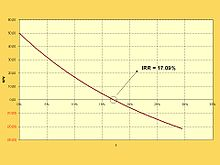IRR: Showing the position of the IRR on the graph of NPV(r) (r is labelled ‘i’ in the graph).

The internal rate of return on an investment or project is the “annualized effective compounded return rate” or “rate of return” that makes the net present value (NPV as NET*1/(1+IRR)^year) of all cash flows (both positive and negative) from a particular investment equal to zero. In more specific terms, the IRR of an investment is the discount rate at which the net present value of costs (negative cash flows) of the investment equals the net present value of the benefits (positive cash flows) of the investment.

IRR calculations are commonly used to evaluate the desirability of investments or projects. The higher a project’s IRR, the more desirable it is to undertake the project. Assuming all projects require the same amount of up-front investment, the project with the highest IRR would be considered the best and undertaken first. A firm (or individual) should, in theory, undertake all projects or investments available with IRRs that exceed the cost of capital. Investment may be limited by availability of funds to the firm and/or by the firm’s capacity or ability to manage numerous projects.

Calculating the IRR

Given a collection of pairs (time, cash flow), a rate of return for which the net present value is zero is an internal rate of return.

Learning Objectives

Calculate a project’s internal rate of return

Key Takeaways

Key Points

• Given the ( period, cash flow ) pairs (n, Cn) where n is a positive integer, the total number of periods N, and the net present value NPV, the internal rate of return is given by the function in which NPV = 0.
• Any fixed time can be used in place of the present (e.g., the end of one interval of an annuity); the value obtained is zero if and only if the NPV is zero.
• If the IRR is greater than the cost of capital, accept the project. If the IRR is less than the cost of capital, reject the project.

Key Terms

• cost of capital: the rate of return that capital could be expected to earn in an alternative investment of equivalent risk
• net present value profile: a graph of the sum of all cash inflows and outflows adjusted for the time value of money at different discount rates

Given a collection of pairs (time, cash flow) involved in a project, the internal rate of return follows from the net present value as a function of the rate of return. A rate of return for which this function is zero is an internal rate of return.

Given the (period, cash flow) pairs (n, Cn) where n is a positive integer, the total number of periods N, and the net present value NPV, the internal rate of return is given by r in: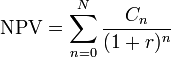Calculating IRR: NPV formula with r as IRR

The period is usually given in years, but the calculation may be made simpler if r is calculated using the period in which the majority of the problem is defined (e.g., using months if most of the cash flows occur at monthly intervals) and converted to a yearly period thereafter. Any fixed time can be used in place of the present (e.g., the end of one interval of an annuity); the value obtained is zero if and only if the NPV is zero.

For example, if an investment may be given by the sequence of cash flows: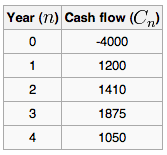Calculating IRR: Cash flows and time

Because the internal rate of return on an investment or project is the “annualized effective compounded return rate” or “rate of return” that makes the net present value of all cash flows (both positive and negative) from a particular investment equal to zero, then the IRR r is given by the formula: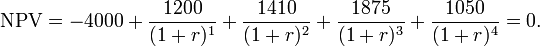Calculating IRR: IRR is the rate at which NPV = 0.

In this case, the answer is 14.3%. If the IRR is greater than the cost of capital, accept the project. If the IRR is less than the cost of capital, reject the project.

The IRR method is easily understood, it recognizes the time value of money, and compared to the NPV method is an indicator of efficiency.

Learning Objectives

Describe the advantages of using the internal rate of return over other types of capital budgeting methods

Key Takeaways

Key Points

• The IRR method is very clear and easy to understand. An investment is considered acceptable if its internal rate of return is greater than an established minimum acceptable rate of return or cost of capital.
• The IRR method also uses cash flows and recognizes the time value of money.
• The internal rate of return is a rate quantity, an indicator of the efficiency, quality, or yield of an investment.

Key Terms

• capital budgeting: The budgeting process in which a company plans its capital expenditure (the spending on assets of long-term value).
• cost of capital: the rate of return that capital could be expected to earn in an alternative investment of equivalent risk

The internal rate of return (IRR) or economic rate of return (ERR) is a rate of return used in capital budgeting to measure and compare the profitability of investment. IRR calculations are commonly used to evaluate the desirability of investments or projects. The higher a project’s IRR, the more desirable it is to undertake the project.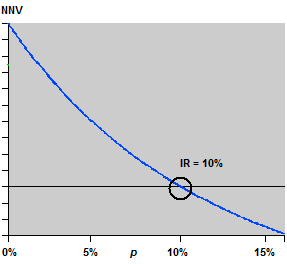Internal rate of return: Internal rate of return is the rate at which the NPV of an investment equals 0.

One advantage of the IRR method is that it is very clear and easy to understand. Assuming all projects require the same amount of up-front investment, the project with the highest IRR would be considered the best and undertaken first. A firm (or individual) should, in theory, undertake all projects or investments available with IRRs that exceed the cost of capital. In other words, an investment is considered acceptable if its internal rate of return is greater than an established minimum acceptable rate of return or cost of capital. Most analysts and financial managers can understand the opportunity costs of a company. If the IRR exceeds this rate, then the project provides financial accretion. However, if the rate of an investment is projected to be below the IRR, then the investment would destroy company value. IRR is used in many company financial profiles due its clarity for all parties.

The IRR method also uses cash flows and recognizes the time value of money. Compared to payback period method, IRR takes into account the time value of money. This is because the IRR method expects high interest rate from investments.

In addition, the internal rate of return is a rate quantity, it is an indicator of the efficiency, quality, or yield of an investment. This is in contrast with the net present value, which is an indicator of the value or magnitude of an investment.

IRR can’t be used for exclusive projects or those of different durations; IRR may overstate the rate of return.

Learning Objectives

Describe the disadvantages of using IRR for capital budging purposes

Key Takeaways

Key Points

• The first disadvantage of IRR method is that IRR, as an investment decision tool, should not be used to rate mutually exclusive projects, but only to decide whether a single project is worth investing in.
• IRR overstates the annual equivalent rate of return for a project whose interim cash flows are reinvested at a rate lower than the calculated IRR.
• IRR does not consider cost of capital; it should not be used to compare projects of different duration.
• In the case of positive cash flows followed by negative ones and then by positive ones, the IRR may have multiple values.

Key Terms

• mutually exclusive: Describing multiple events or states of being such that the occurrence of any one implies the non-occurrence of all the others.
• duration: A measure of the sensitivity of the price of a financial asset to changes in interest rates, computed for a simple bond as a weighted average of the maturities of the interest and principal payments associated with it

The first disadvantage of the IRR method is that IRR, as an investment decision tool, should not be used to rate mutually exclusive projects but only to decide whether a single project is worth investing in. In cases where one project has a higher initial investment than a second mutually exclusive project, the first project may have a lower IRR (expected return), but a higher NPV (increase in shareholders ‘ wealth) and should thus be accepted over the second project (assuming no capital constraints).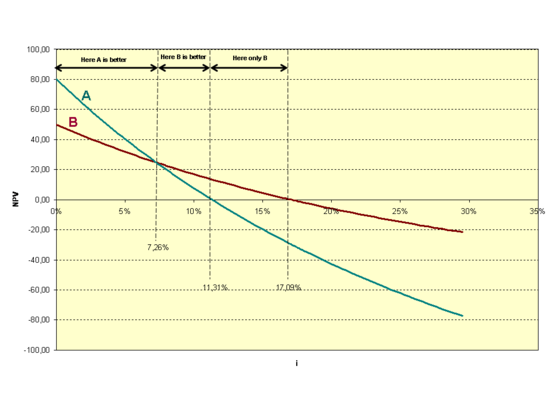Disadvantage of IRR: NPV vs discount rate comparison for two mutually exclusive projects. Project A has a higher NPV (for certain discount rates), even though its IRR (= x-axis intercept) is lower than for project B

In addition, IRR assumes reinvestment of interim cash flows in projects with equal rates of return (the reinvestment can be the same project or a different project). Therefore, IRR overstates the annual equivalent rate of return for a project whose interim cash flows are reinvested at a rate lower than the calculated IRR. This presents a problem, especially for high IRR projects, since there is frequently not another project available in the interim that can earn the same rate of return as the first project. When the calculated IRR is higher than the true reinvestment rate for interim cash flows, the measure will overestimate–sometimes very significantly–the annual equivalent return from the project. The formula assumes that the company has additional projects, with equally attractive prospects, in which to invest the interim cash flows.

Moreover, since IRR does not consider cost of capital, it should not be used to compare projects of different duration. Modified Internal Rate of Return (MIRR) does consider cost of capital and provides a better indication of a project’s efficiency in contributing to the firm’s discounted cash flow.

Last but not least, in the case of positive cash flows followed by negative ones and then by positive ones, the IRR may have multiple values.

Multiple IRRs

When cash flows of a project change sign more than once, there will be multiple IRRs; in these cases NPV is the preferred measure.

Learning Objectives

Explain the best way to evaluate a project that has multiple internal rates of return

Key Takeaways

Key Points

• In the case of positive cash flows followed by negative ones and then by positive ones, the IRR may have multiple values.
• It has been shown that with multiple internal rates of return, the IRR approach can still be interpreted in a way that is consistent with the present value approach provided that the underlying investment stream is correctly identified as net investment or net borrowing.
• NPV remains the “more accurate” reflection of value to the business. IRR, as a measure of investment efficiency may give better insights in capital constrained situations. However, when comparing mutually exclusive projects, NPV is the appropriate measure.

Key Terms

• Modified Internal Rate of Return: The modified internal rate of return (MIRR) is a financial measure of an investment’s attractiveness. It is used in capital budgeting to rank alternative investments of equal size. As the name implies, MIRR is a modification of the internal rate of return (IRR) and, as such, aims to resolve some problems with the IRR.
• mutually exclusive: Describing multiple events or states of being such that the occurrence of any one implies the non-occurrence of all the others.

In the case of positive cash flows followed by negative ones and then by positive ones, the IRR may have multiple values. In this case a discount rate may be used for the borrowing cash flow and the IRR calculated for the investment cash flow. This applies for example when a customer makes a deposit before a specific machine is built.

In a series of cash flows like (−10, 21, −11), one initially invests money, so a high rate of return is best, but then receives more than one possesses, so then one owes money, so now a low rate of return is best. In this case it is not even clear whether a high or a low IRR is better. There may even be multiple IRRs for a single project, like in the above example 0% as well as 10%. Examples of this type of project are strip mines and nuclear power plants, where there is usually a large cash outflow at the end of the project.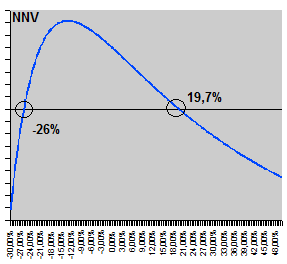Multiple internal rates of return: As cash flows of a project change sign more than once, there will be multiple IRRs. NPV is a preferable metric in these cases.

When a project has multiple IRRs, it may be more convenient to compute the IRR of the project with the benefits reinvested. Accordingly, Modified Internal Rate of Return (MIRR) is used, which has an assumed reinvestment rate, usually equal to the project’s cost of capital.

It has been shown that with multiple internal rates of return, the IRR approach can still be interpreted in a way that is consistent with the present value approach provided that the underlying investment stream is correctly identified as net investment or net borrowing.

Despite a strong academic preference for NPV, surveys indicate that executives prefer IRR over NPV. Apparently, managers find it easier to compare investments of different sizes in terms of percentage rates of return than by dollars of NPV. However, NPV remains the “more accurate” reflection of value to the business. IRR, as a measure of investment efficiency may give better insights in capital constrained situations. However, when comparing mutually exclusive projects, NPV is the appropriate measure.

Modified IRR

The MIRR is a financial measure of an investment’s attractiveness; it is used to rank alternative investments of equal size.

Learning Objectives

Calculate a project’s modified internal rate of return

Key Takeaways

Key Points

• MIRR is a modification of the internal rate of return (IRR) and as such aims to resolve some problems with the IRR.
• More than one IRR can be found for projects with alternating positive and negative cash flows, which leads to confusion and ambiguity. MIRR finds only one value.
• MIRR = {[FV(positive cash flows, reinvestment rate )/-PV(negative cash flows, finance rate)]^(1/n)}-1.

Key Terms

• cost of capital: the rate of return that capital could be expected to earn in an alternative investment of equivalent risk
• reinvestment rate: The annual yield at which cash flows from an investment can be reinvested.

The modified internal rate of return (MIRR) is a financial measure of an investment ‘s attractiveness. It is used in capital budgeting to rank alternative investments of equal size. As the name implies, MIRR is a modification of the internal rate of return (IRR) and as such aims to resolve some problems with the IRR.

While there are several problems with the IRR, MIRR resolves two of them. Firstly, IRR assumes that interim positive cash flows are reinvested at the same rate of return as that of the project that generated them. This is usually an unrealistic scenario and a more likely situation is that the funds will be reinvested at a rate closer to the firm’s cost of capital. The IRR therefore often gives an unduly optimistic picture of the projects under study. Generally, for comparing projects more fairly, the weighted average cost of capital should be used for reinvesting the interim cash flows. Secondly, more than one IRR can be found for projects with alternating positive and negative cash flows, which leads to confusion and ambiguity. MIRR finds only one value.

MIRR is calculated as follows: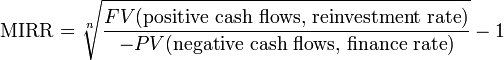MIRR: The formula for calculating MIRR.

Where n is the number of equal periods at the end of which the cash flows occur (not the number of cash flows), PV is present value (at the beginning of the first period), and FV is future value (at the end of the last period).

The formula adds up the negative cash flows after discounting them to time zero using the external cost of capital, adds up the positive cash flows including the proceeds of reinvestment at the external reinvestment rate to the final period, and then works out what rate of return would cause the magnitude of the discounted negative cash flows at time zero to be equivalent to the future value of the positive cash flows at the final time period.

Let take a look at one example. If an investment project is described by the sequence of cash flows: Year 0: -1000, year 1: -4000, year 2: 5000, year 3: 2000. Then the IRR is given by: NPV = -1000 – 4000 * (1+r)-1 + 5000*(1+r)-2 + 2000*(1+r)-3 = 0. IRR can be 25.48%, -593.16% or -132.32%.

To calculate the MIRR, we will assume a finance rate of 10% and a reinvestment rate of 12%. First, we calculate the present value of the negative cash flows (discounted at the finance rate): PV(negative cash flows, finance rate) = -1000 – 4000 *(1+10%)-1 = -4636.36.

Second, we calculate the future value of the positive cash flows (reinvested at the reinvestment rate): FV (positive cash flows, reinvestment rate) = 5000*(1+12%) +2000 = 7600.

Third, we find the MIRR: MIRR = (7600/4636.36)(1/3) – 1 = 17.91%.